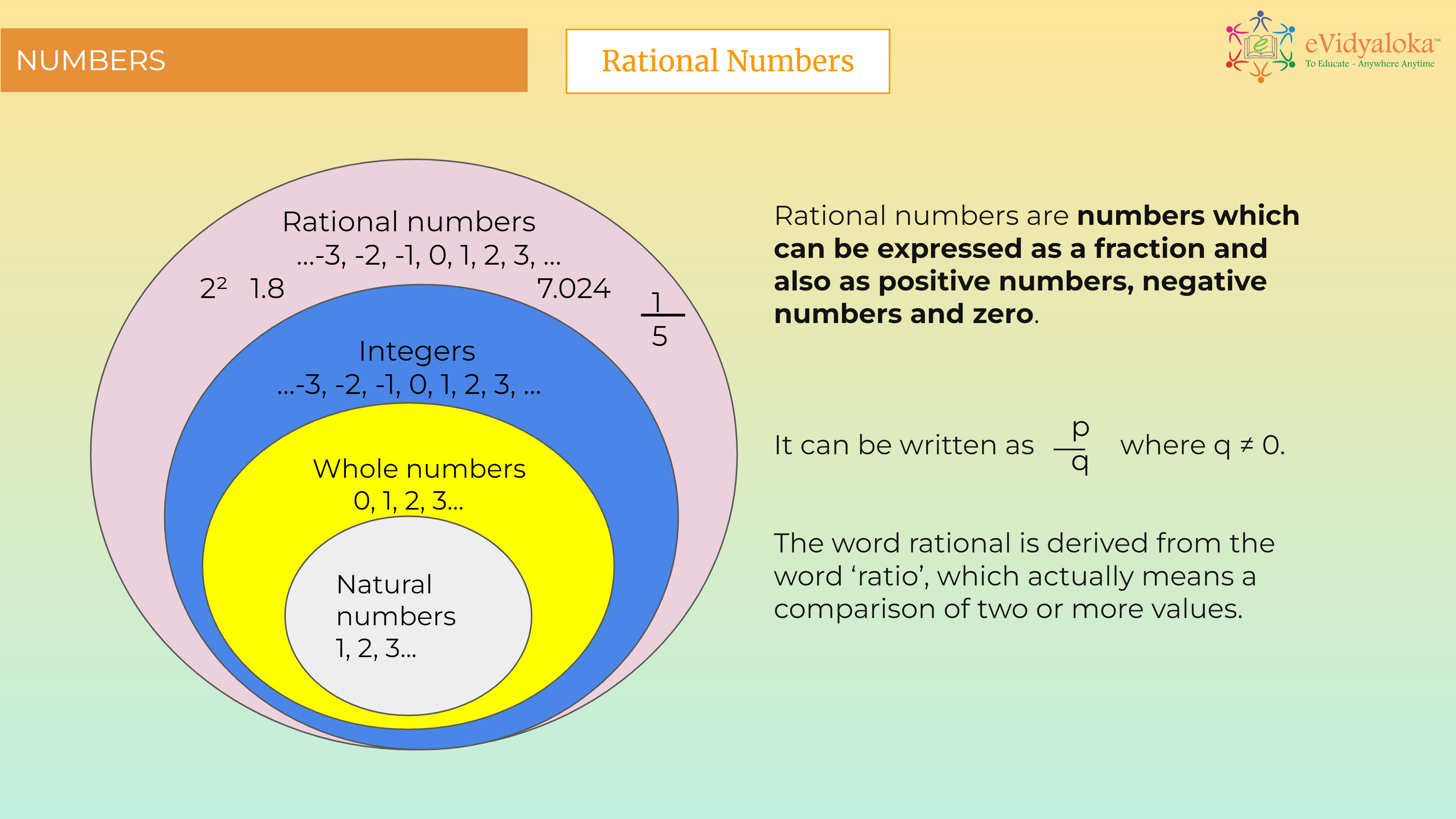# Rational Numbers

``````

Dear Volunteer Teacher,

Kindly teach the bridge concept of Rational Numbers prior to teaching the related textbook chapters.
Thank you.

## Course Content

• Definition
• Representing rational numbers on a number line
• Rational numbers between two rational numbers
• Comparing rational numbers
• Ordering rational numbers
• Properties of rational numbers

## Learning Outcomes

Learning outcomes has been listed below which will serve as your guide for what students need to learn.

SWBAT:

• understand Rational numbers and represet the same on a number line.
• find numbers berween 2 rational numbers and compare and order them.
• They will have an idea of all the properties and identity and inverse concepts of rational numbers.

## PPT Deck

To make the lesson come alive, please find below PPT deck